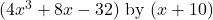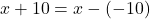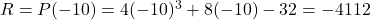## What is the remainder when 4x^3+8x-32 is divided by x+10?

Question

What is the remainder when 4x^3+8x-32 is divided by x+10?

in progress 0
5 months 2021-09-05T11:54:58+00:00 1 Answers 0 views 0

The remainder is -4112.

Step-by-step explanation:

We can use the Polynomial Remainder Theorem. According to the PRT, when we divide a polynomial P(x) by a binomial in the form of (x – a), then the remainder will be P(a).

We are dividing:We can rewrite the divisor as:So, a = -10.

Then according to the PRT, the remainder of the operation will be: